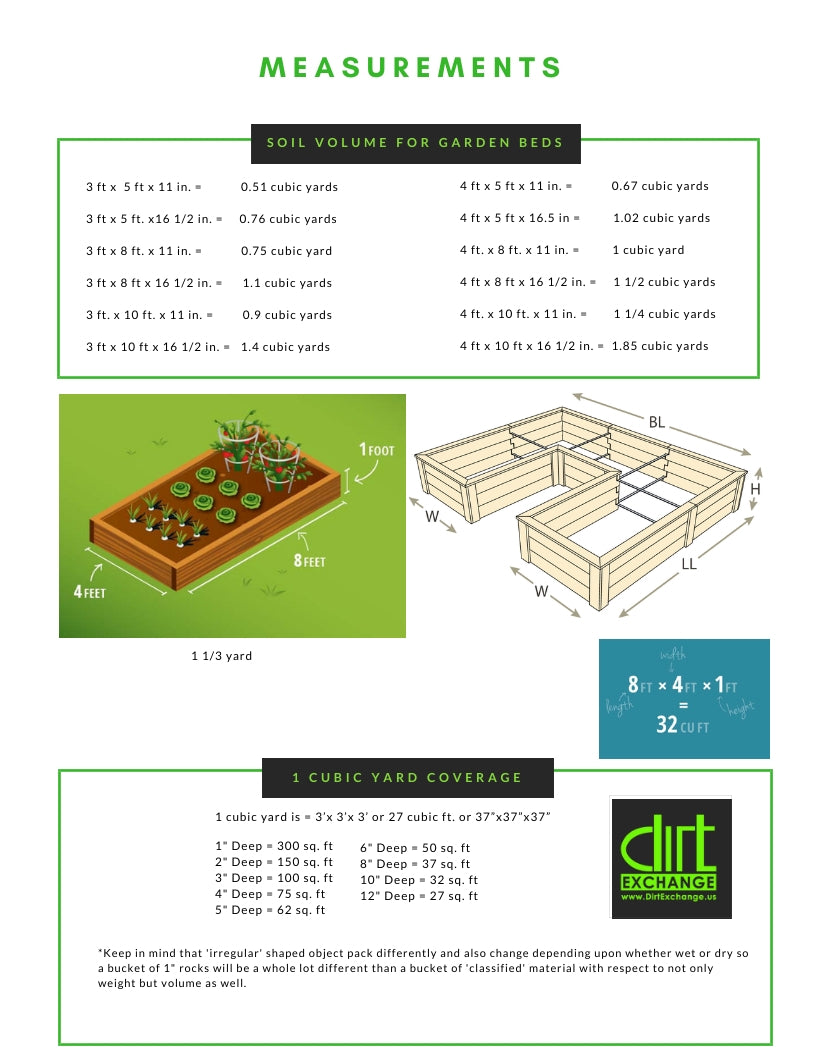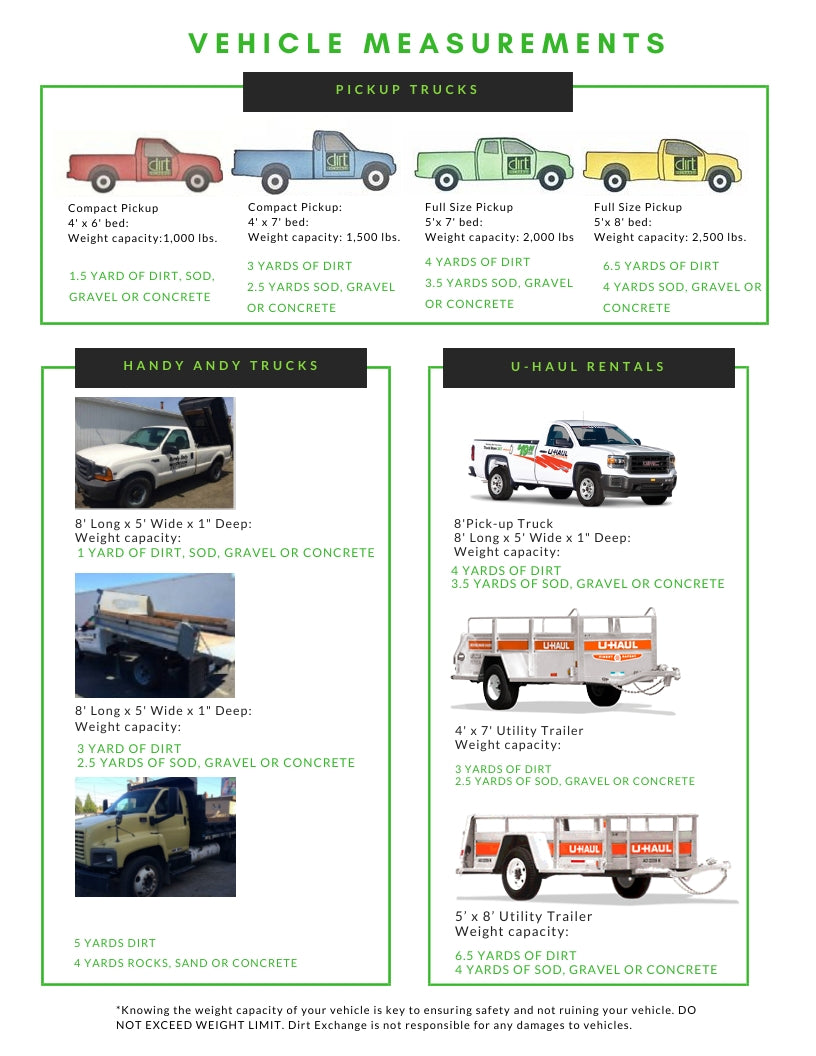# Yardage, Measurements, and AutomobilesCheck out these pages for common soil measurements:

#### •   Formulas and How to determine how many yards you needContainer Measurements:AUTOMOBILE MEASUREMENTS:To determine how many yards you need:

First, choose the right material.
Before you start your project, you need to first ensure you’re choosing the right material for your specific project. Certain products are better for certain applications. You can contact us here to ask questions or check out our product descriptions for more help.

Second, measure once then measure again.
In order to determine how much material you will need, you need to know the size of the area you need filled. This requires you to measure the width, length, and depth. Once you have these numbers, be sure to write them down. Or draw it out on paper as accurately as possible to give you a good visual aid. Depending on the size of the area, you may want to use a measuring wheel instead of a measuring tape, and you may want to get someone to help you.  When ordering, you need to be certain that you will have the right amount of material. Too little leaves bare spots, and if you purchase an excess, you will have wasted money. Accurately calculate the amount of material you need from your yard's measurements to ensure you buy what you need.

Convert.

How to get cubic yards? Measure by inches, convert to cubic ft, to cubic yards.

When you do the math, you need to ensure that your measurements are in the same units. To make it easy on you, always try to take your measurements in feet. If any area of your project is less than one foot, you need to convert the measurement from inches to feet, which you can do by dividing that number by 12. So, for example, if you had a depth of 6 inches, you would convert it to feet by dividing 6 by 12. So 6 ÷ 12 = .5 feet. Calculate the cubic feet.
Once all your measurements are in feet, you can easily calculate the total cubic feet of your project. This is done by multiplying the length x width x depth. If you have a 10’-wide x 20’-long driveway that’s .5’ deep, you would multiply 10 x 20 x .5 for a total of 100 cubic feet.  Convert to cubic yards.
Since gravel is measured in cubic yards, you’ll need to convert your measurement from cubic feet to cubic yards. You can do this by dividing the total number by 27. In terms of the example above, your math would look like this: 100 ÷ 27 = 3.7, or 3.7 cubic yards. While this math may be easy for you to do on your own, you can also use our handy material calculator on any of our product pages to receive the most accurate measurement for your project. If your number comes out as a fraction -- and it probably will -- round up. In the example above, you would round the 3.7 cubic yards of crushed stone to 4 cubic yards of crushed stone. It is better to have a little extra than to run short.

Area formulas:

How to determine the area of a CIRCLE:
Multiply pi ( = 3.14) by the square of the radius, If you know the diameter, the radius is 1/2 of the diameter.

How to determine the area of a RECTANGLE or PARALLELOGRAM:
Multiply the base times the height.

How to determine the area of a TRIANGLE:
Multiply the base times one-half the height.

How to determine the area of a TRAPEZOID:
Add the lengths of the 2 parallel sides, divide by 2 to get the average length of the parallel sides. Multiply this by the height (distance between the parallel sides).